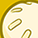# Power Management ICs Forum DiscussionsLevel 1Level 1

# Doubt on max power at the Inductor (10uH) connected between VCC1 and BCKSW for TLE9471-3ES V33

Hi,

I would like to know the maximum power at the inductor(L2)(The inductor value is 10uH) that we have taken as per the datasheet recommended value, considering that the VCC1 is having the max voltage and it is sensed across the BCKSW and current at VCC1 is max, what is the maximum power that we get across this L2, Considering the inductor power dissipation, The inductor DCR value is 0.18ohm and L2=10uHModeratorModerator

# Re: Doubt on max power at the Inductor (10uH) connected between VCC1 and BCKSW for TLE9471-3ES V33

Hello,

There are three parts to the inductor losses, core loss, DC resistance loss and AC resistance loss.
Losses can be calculated based on the below formula

P_loss_Inductor = Pcore + Pdcr + Pacr

Core loss
Pcore (mW) = K1 * f^x * B^y * Ve

Where:
K1 = Constant for core material
f = Frequency in kHz
B = Peak Flux Density in kGauss
x = Frequency exponent
y = Flux Density exponent
Ve = Effective core volume (cm3)

Dc resistance loss
Pdcr (W) = Irms^2 * DCR

Where:
Irms = The rms value of the peak current applied to
the inductor
DCR = The dc resistance of the inductor

AC resistance loss
Pacr (W) = Irms2 × ACR

Where:
Irms = The rms value of the peak-peak ripple current
applied to the inductor
ACR = The ac resistance of the inductor

Inductor constants should be available in the inductor datasheet.
Hint,
AC winding resistance values are not always readily available from magnetics vendors. AC resistance loss is less compared to other two components, Hence initial calculations can be with only core loss and DC resistance loss.

Regards

Vishnu